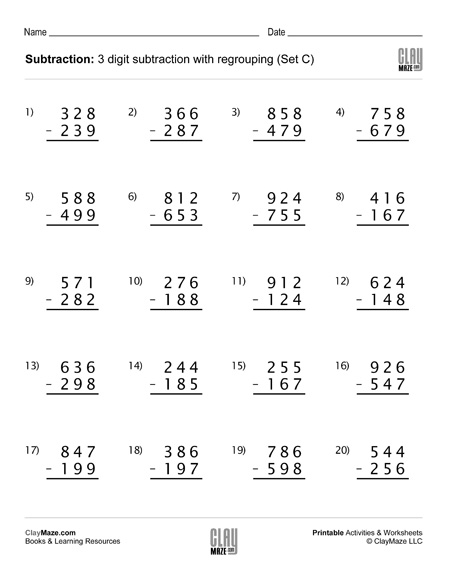# Subtraction Worksheet – 3 Digit Subtraction with Regrouping (Set C)

Use this 3 digit subtraction worksheet with regrouping for lots of practice. There are 20 problems and numbers have enough spacing between the digits for borrowing.